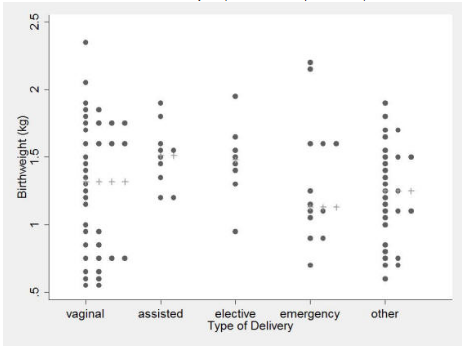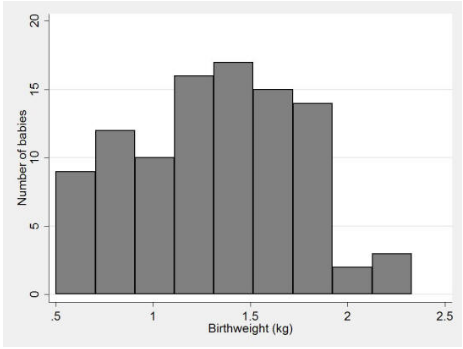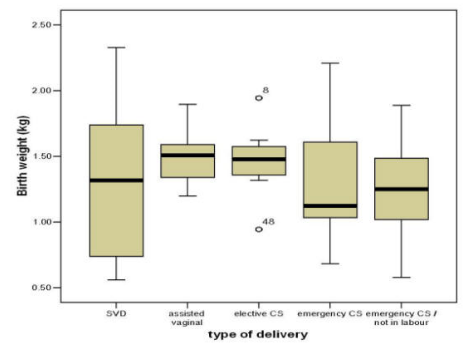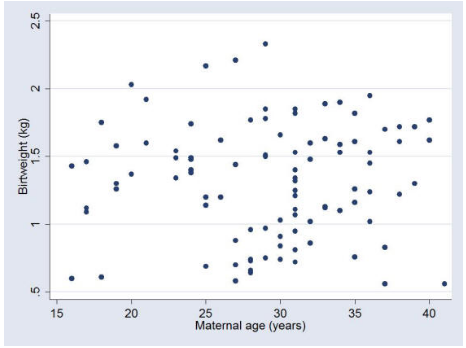# Graphical methods in Statistics

## Graphical methods in Statistics

### Statistics: Graphical Methods

This section covers:

•        Dot plots
•        Histograms
•        Box-whisker plots
•        Scatter plots
•        Bar charts
•        Pie charts

A picture is worth a thousand words, or numbers, and there is no better way of getting a 'feel' for the data than to display them in a figure or graph. The general principle should be to convey as much information as possible in the figure, with the constraint that the reader is not overwhelmed by too much detail.

Dot Plots

The simplest method of conveying as much information as possible is to show all of the data and this can be conveniently carried out using a Dot plot.

Example

Data on birth weight and type of delivery are shown in Figure 1 as a Dot plot. This method of presentation retains the individual subject values and clearly demonstrates differences between the groups in a readily appreciated manner. An additional advantage is that any outliers will be detected by such a plot. However, such presentation is not usually practical with large numbers of subjects in each group because the dots will obscure the details of the distribution.

Figure 1 Dot plot showing birth weight of 98 babies by type of delivery with the medians shown by '+' (data from Simpson 2004)

###Histograms

The patterns may be revealed in a large data set of a numerically continuous variable by forming a histogram. This is constructed by first dividing up the range of the variable into several non-overlapping and equal intervals (also called “classes” or “bins”), then counting the number of observations in each. A histogram for all the 98 birth weights in the Simpson (2004) data is shown in Figure 2. The area of each histogram block is proportional to the number of subjects in the particular birth-weight category concentration group. Thus, the total area in the histogram blocks represents the total number of volunteers. Relative frequency histograms, where the y-axis shows the proportion of the observations in each bin rather than an absolute number, allow comparison between histograms made up of different numbers of observations which may be useful when studies are compared.

Figure 2 Histogram of birth weight of 98 babies (data from Simpson 2004)The choice of the number of intervals is important. Too few intervals and much important information may be smoothed out; too many intervals and the underlying shape will be obscured by a mass of confusing detail. It is usual to choose between 5 and 15 intervals, but the correct choice will be based partly on a subjective impression of the resulting histogram. Histograms with bins of unequal interval length can be constructed but they are usually best avoided.

Advantages of histograms include the ability to visualise the shape of the frequency distribution and to demonstrate central tendency. However, because data are grouped into intervals, exact values of each observation cannot be determined.

Box-Whisker Plot

If the number of points is large, a Dot plot can be replaced by a box-whisker plot which is more compact than the corresponding histogram. Such a plot is illustrated in Figure 3 for the birth weight and type of delivery from Simpson (2004).

Figure 3 Box-Whisker plot of birth weight of babies by method of delivery (data from Simpson, 2004)The 'whiskers' in the diagram indicate the minimum and maximum values of the variable under consideration. The median value is indicated by the central horizontal line while the lower and upper quartiles by the corresponding horizontal ends of the box. The box-whisker plot as used here therefore displays the median and two measures of spread, namely the range and interquartile range. Any skew in the data will also be apparent, as determined from the location of the median in relation to the lower and upper quartiles. A variant of the box-whisker, which shows outliers, is to define a range and only extend the whiskers to the extreme values of the range. If we define the width of the IQR as the hinge, where the hinge is the upper quartile minus the lower quartile  then the outlier range is

Lower quartile - 1.5xhinge to Upper quartile + 1.5xhinge.

Thus in Figure 3, we can see that, except for the group elective C5,  all points are within the outlier range and the whiskers extend to the minimum and maximum values. For the group elective C5 the whiskers extend only a short distance from the lower and upper quartiles and these are the extreme points in the outlier range. The two points labelled 8 and 48 are outside of this range.

Boxplots are especially helpful in visually comparing two or more groups.

Scatterplots

When one wishes to show a relationship between two continuous variables, a scatterplot can be employed. Figure 4 shows a scatterplot of birthweight by maternal age.

Figure 4 Scatterplot of birth weight by maternal age (Simpson 2004)It is apparent from this scatterplot that birthweight and maternal age are not associated in this sample. From a graphical perspective, if one variable x could be the cause of another variable y, then it is conventional to plot the x variable on the horizontal axis and the y variable on the vertical axis. In this case, as maternal age could theoretically influence birthweight, but not vice versa, then maternal age is plotted on the x-axis and birthweight on the y-axis.

Advantages of scatterplots are that they can demonstrate associations between two variables, they retain the exact data values (including minimum and maximum values), and that they may make outliers apparent. However, it can be hard to visualise individual results where data sets are very large, and weak relationships may not be apparent.

Bar Charts and Pie Charts

Bar charts and pie charts can be used to display categorical data.

The heights of bars in a bar chart represent the frequencies (or relative frequencies) in each group. Note that in a bar chart, there is a gap between each bar. This is unlike a histogram, where there are no gaps between the bars, reflecting the continuous nature of the underlying variable.

In a pie chart, a circle is divided into slices, such that each slice represents a different category and the size of the slice is proportional to the relative frequency of that category. Conventionally, the categories in a pie chart are ordered clockwise from the largest slice to the smallest, starting at the 12 o’clock position. In general, pie charts are best avoided, especially if there are a large number of categories (say >5). If they are used, the relative frequency (given as a percentage) should be given for each category, as they can be difficult to estimate visually.

References

• Campbell MJ, Machin D and Walters SJ. Medical Statistics: a Commonsense Approach 4th ed. Chichester: Wiley-Blackwell 2007
• Simpson A. PhD Thesis Institute of Primary Care, University of Sheffield, 2004
• Freeman JV, Walters SJ and Campbell MJ (2008) How to display data. Oxford BMJ Books, Blackwell publishing

© MJ Campbell 2016, S Shantikumar 2016